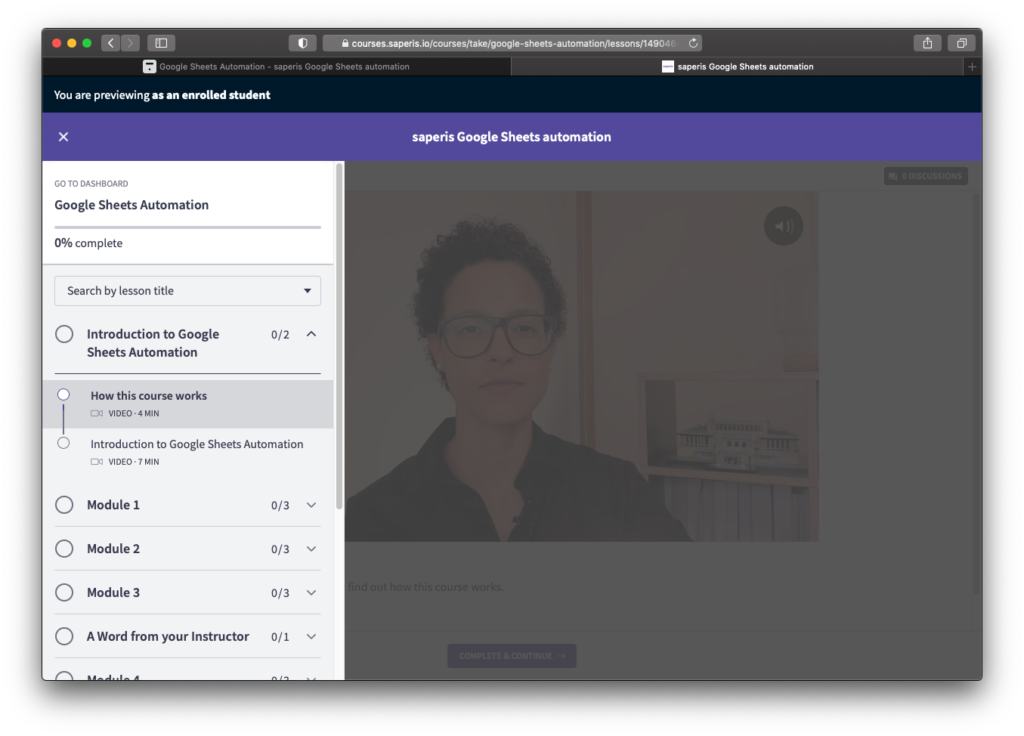# Google Sheets If Then Formula – What it is and how to use it

Google Sheets If Then formula allows us to react in two different ways depending on if something is true or false. Learn how to use this formula now!

# Google Sheets If Then Formula – What it is and how to use it

The If Then formula in any spreadsheet program is super useful if you’re conducting a logical test. In this post we want to dive into Google Sheets If Then formula and see how to use it.

This tutorial also comes in video form if you prefer to watch me show and explain every step of using Google Sheets If Then formula.

## What is a logical test?

Before we get to actually using Google Sheets If Then formula, let’s have a look at what it is and why you would want to use it.

A logical test returns one value if a logical expression is TRUE and another if it is FALSE. Here a real life example: If a sales rep reaches her targeted sales revenue, then that sales rep gets a bonus. So in a spreadsheet we would want to check for a logical expression (has the sales rep reached the target?) and give back TRUE if the sales rep was successful or FALSE if she didn’t reach the target.

Maybe a second example to drive the principal of logical test home. A school keeps a list of all the student grades. If a student has straight A’s in all courses (if grades = A) then that student receives an award (TRUE). If not (FALSE) the students gets a letter with encouragement to keep up the good work.

## Google Apps Script Online Course

Learn how to automate Google Sheets with Google Apps Script! Check out our comprehensive online course.## How to use the If Then Formula in Google Sheets

We need something to compare a.k.a. the logical expression. In our example we’re comparing if the actual revenue (column C) is higher than or equal the forecasted revenue (column B). If that is the case (TRUE), we write out Yes in column D. If that is not the case (FALSE), we write out No in column D.

The way you actually write this in Google Sheets is as follows: `=IF(C2>=B2, "Yes", "No")`. And if you want to use this logical test for the following rows you simply double click on the bottom right corner of the cell D2, which contains our If Then formula.

By the way, in most spreadsheet applications we use the word functions for formulas. This is programming speak for formula. You can read more about Google Sheets If Then function in this official Google support article.